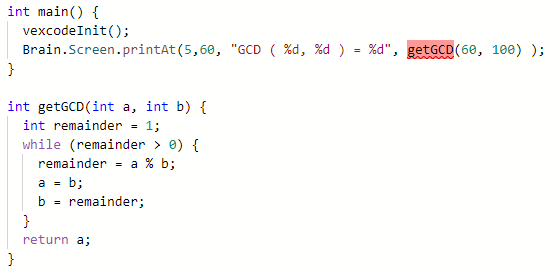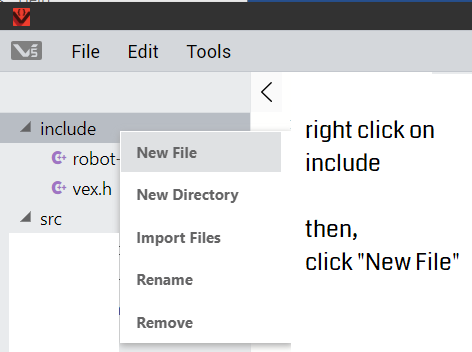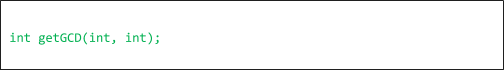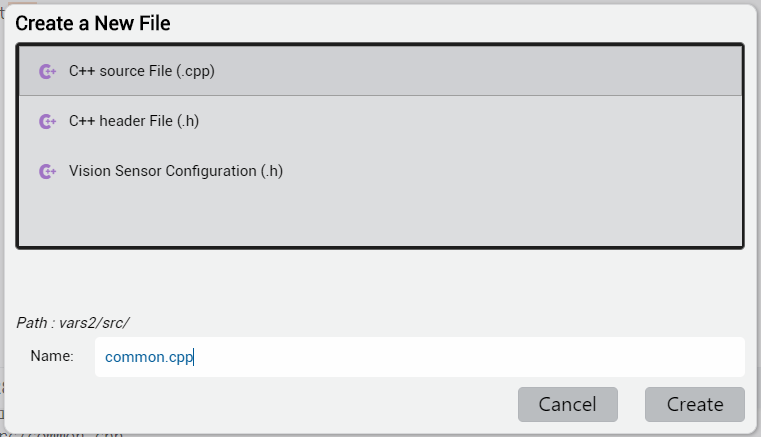# Syntax to Create Functions - Programming

## Function definition

Now that we have gone through a few samples, let’s look at the general form of a function definition:

A function returns no special value, nor no incoming parameters.

```void function-name() {

Declarations of variables, etc.
Expressions...
//no explicit return is necessary.
}
```

A function returns no special value, but with one parameter.

```void function-name(data-type variable) {

Declarations of variables, etc.
Expressions...
//no explicit return is necessary.
}

If you want multiple variables, you simply use "," as a delimitor:
```

A function returns no special value, but with more than one parameter.

```void function-name(data-type variable, data-type variable, etc.) {

Declarations of variables, etc.
Expressions...
//no explicit return is necessary.
}
```

A function returns a value of a specific datatype, and with more than input one parameter.

```void function-name(data-type variable, data-type variable, etc.) {

Declarations of variables, etc.
Expressions...
return return-value
}
```

The return type of a function must be the same as the data type of the return-value that function returns. The return-value can be a constant value or a variable. It must be preceded with the keyword “return.

The following rules govern the return type:

• There are almost no restrictions on the return type, except array. (Array is another more advanced topic regarding how to create your data. It is outside the scope of this article and not be covered here.
• Specifying that the return type “void” means no return value is necessary. The clause “return” is implicit. That means you have to explicitly state “return” at the end of a “void” function.

## Creating a function before the main() or after?

The compiler reads your file from top to bottom. Thus, the order does matter.

Let’s take the simple Greatest Common Denominator (GCD) function as an example. You will get an error: `use of undeclared identifier 'getGCD'`Actually this is true whether it is from the main( ) or other calling function block, i.e. the “called function block” must precede the “calling function block.”

Two possible solutions:

1. Move the “called function” above the “calling function block,” in the example "main()."
```int getGCD(int a, int b) {
int remainder = 1;
while (remainder > 0) {
remainder = a % b;
a = b;
b = remainder;
}
return a;
}

int main() {
Brain.Screen.printAt(5,60, “GCD ( %d, %d ) = %d”, getGCD(60, 100) );
}
```
2. Put the prototype (also called signature) of the function before the “calling function block.”
```int getGCD(int, int);

int main() {
Brain.Screen.printAt(5,60, "GCD ( %d, %d ) = %d", getGCD(60, 100) );
}

int getGCD(int a, int b) {
int remainder = 1;
while (remainder > 0) {
remainder = a % b;
a = b;
b = remainder;
}
return a;
}
```

## What if you wish to modularize functions in different files?

##### Step 1: Create a header file, and put the prototype of the function into this file.

e.g. create a file named “myFuncs.h”

You need to “add” this header file into your project before compiling your code. This allows the VEXcode V5 Text IDE “to be aware of” the inclusion of this new header file before it starts building your project.

Here is how:In this header file “common.h” (you can name whatever you want, as long as it is alphanumeric with no space.)##### Step 2: Create a separate cpp file, e.g., called common.cppMove the function getGCD(...) into this file:

```//this is the common.cpp file

int getGCD(int a, int b) {
int remainder = 1;
while (remainder > 0) {
remainder = a % b;
a = b;
b = remainder;
}
return a;
}
```

Now, all you have in main cpp file will consist of the following:

```#include "vex.h"
#include "common.h"
using namespace vex;

int main() {
vexcodeInit();
Brain.Screen.printAt(5,60, "GCD ( %d, %d ) = %d", getGCD(60, 100) );
}
```

Future topics will be covered in more advanced sections of the Knowledge Base in the future:

• Pass by value vs. pass by reference
• Pass in an array
• Pass in a structure